# Making Sense of RF Output Power

In RF testing, the output power level of signal generators is an important factor as they need to maintain spectral purity and level accuracy. The automatic leveling control (ALC) and various combinations of attenuators determine the power range of signal generators. In fact, the output power of signal generators needs to be as low as -120 dBm for receiver sensitivity testing, and the peak power requirement for RF power amplifier testing must be more than +20 dBm.

Although “power” is a pretty basic concept, it is important to understand the kind of ‘’power” you are referring to. Let’s take a closer look at the difference between average power, envelope power, peak envelop power, and measurement applications for high/low output power.

## What is “Power”?

The International System of Units defines the watt (W) as a unit of power – one watt is one joule per second and is used to quantify the rate of energy transfer. At direct current (DC) and low frequencies, voltage and current measurements are simple and straightforward. Power (P) is the product of voltage (V) and current (I).

For low-frequency signals, both voltage and current vary with time. The energy transfer rate (instantaneous power) also varies with time. In Figure 1 below, the instantaneous power shifts around cycles as represented by the blue curve. Average power is calculated by integrating the area under the P curve.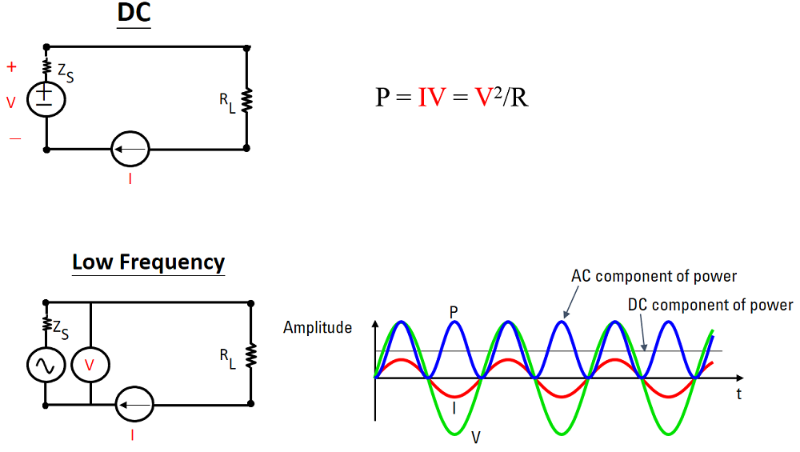Figure 1. DC and low frequencies power measurements

However, as frequency increases, voltage and current measurements become difficult and impractical, so engineers measure power directly. Figure 2 represents three continuous waves (CW) with the same voltage level but different frequencies. Pi (green curve) is instantaneous power and it varies with time, while Pavg (red line) is average power. Notice that average power remains constant and independent of frequency which is a good quantity for high-frequency signals. Let’s take a closer look at different definitions of power for RF measurements.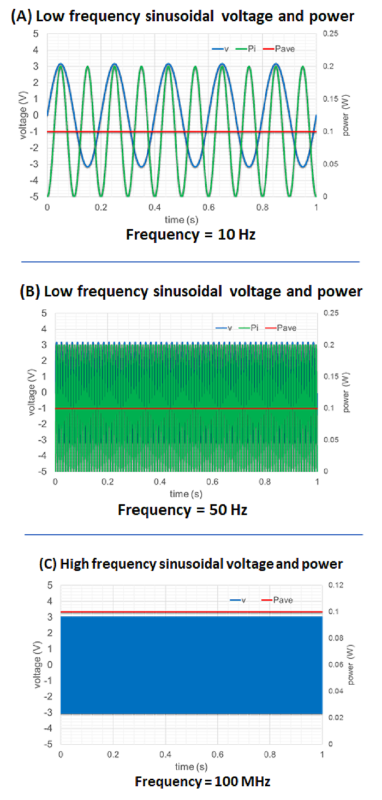Figure 2. Low and high-frequency power measurements

### Average Power

As frequency increases, the impedance will vary. The term “average power” is very popular and is used to specify all RF and microwave systems as the instantaneous power variations are too fast to be meaningful. Average power is the average energy transfer rate across many time periods of the lowest frequency.

### Envelope Power and Peak Envelope Power

For some applications, engineers examine the effects of modulation or transient conditions without examining details of the RF carrier waveform. Figure 3 below illustrates high-frequency modulated signal power measurements. The upper graph represents the voltage envelope of the modulated signal. The lower left graph shows the instantaneous power of the signal in green and the average power in red.

The envelope power is measured by averaging the power over a time period that is long compared to the period of the highest modulation frequency, but short compared to the period of the carrier. The lower right graph shows the envelope power in red. The maximum envelope power is called “peak envelop power (PEP)” which is an important parameter that is used to characterize the output power of a modulated signal.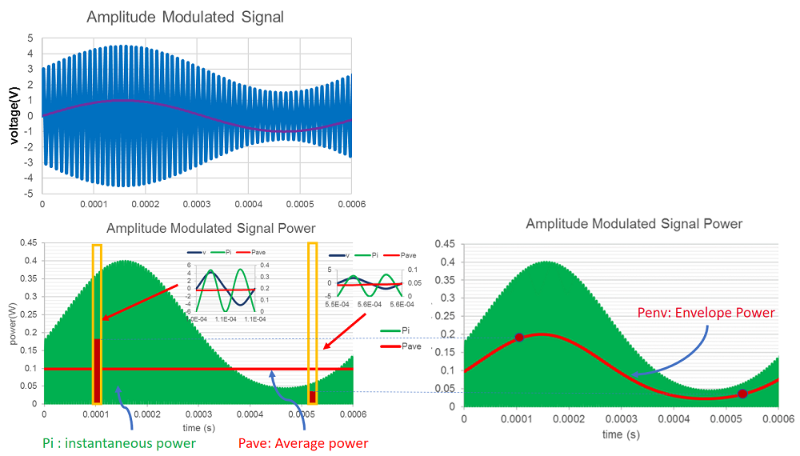Figure 3. Voltage envelope and power envelope of a high-frequency modulated signal

Many of the digitally modulated signals appear noise-like in the time and frequency domain. Power Complementary Cumulative Distribution Function (CCDF) curves help characterize the higher-level power statistics of a digitally-modulated signal. Use the CCDF of a signal generator to identify the peak-to-average ratio (PAR) of a signal waveform as shown in Figure 4. The signal waveform is a 64 QAM and symbol rates are at 1 Msps with a root-raised-cosine (RRC) baseband filter. The peak value is 5.95 dB above the average power. If the output amplitude (average power) set to 0 dBm, the PEP is equal to output amplitude plus PAR, i.e. +5.95 dBm.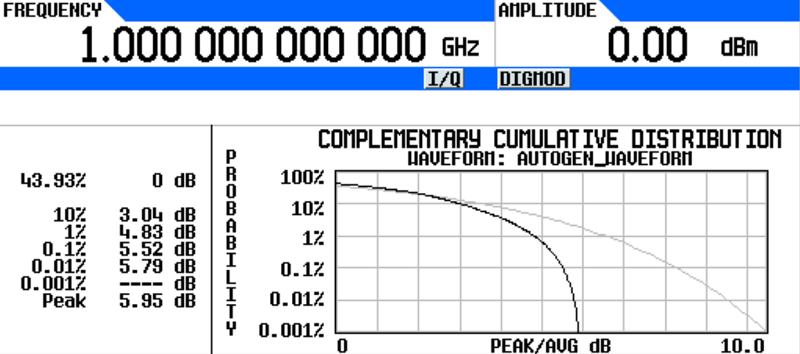Figure 4. CCDF plot from waveform utility of Keysight’s N5182B signal generator

The amplitude level setting on a signal generator cannot be greater than the maximum output power of the signal generator minus the peak-to-average ratio, or it may result in compression of the signal generator’s amplifier. For example, the maximum output power of a signal generator is +18 dBm and the waveform’s PAR is 5.95 dB. The maximum amplitude setting (average power) for the signal generator is +12.05 dBm (18 - 5.95 = 12.05).

## Examples of CW and Modulated Signals

Let's take a look at some examples. In Figure 5 (left panel), there are 3 different settings on the signal generator. The amplitude level is set at 0 dBm, and the frequency is set to 1 GHz.

• A is a CW signal
• B is a QPSK (quadrature phase shift keying) modulated signal with a symbol rate of 1 Msps; it uses an RRC (root-raised-cosine) baseband filter.
• C is a 64-QAM (quadrature amplitude modulation) modulated signal with a symbol rate of 1 Msps; it uses an RRC baseband filter.

The signal analyzer’s measurement results are on the right of Figure 5. The results include “Power vs. Time”, “Mean power” (Average power), and “Peak-to-Mean” (PAR). Cable loss between the signal generator and the signal analyzer is about 1 dB.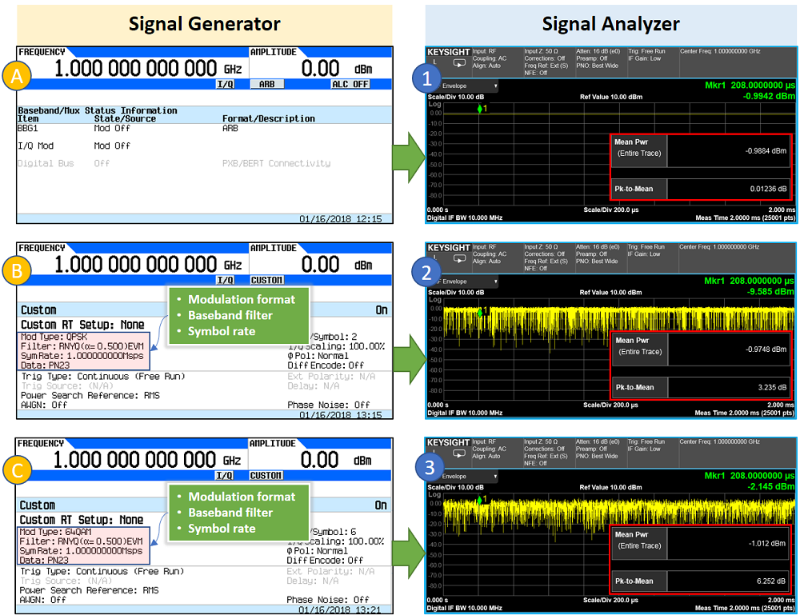Figure 5. Amplitude settings and measurement results of CW and modulation signals

The amplitude setting on a signal generator is “Average Power” (Mean Power for signal analyzer). You will notice that all three outputs’ “Mean Pwr” measurement results are about -1 dBm (due to the cable loss).

The results of “Pk-to-Mean” are different for all three outputs. Result ① has a Pk-to-Mean measurement of almost 0 dB because it’s a CW signal. Results ② & ③ are modulated signals and you can see the power variations in the time domain. A 64-QAM modulation signal has more power variations than a QPSK’s. This means the Pk-to-Mean measurement of the 64-QAM modulation signal is bigger than the QPSK’s. It is important to know the Pk-to-Mean value of the modulation signal before setting the amplitude level on a signal generator to avoid exceeding the maximum output power of the signal generator.

## What Does Specification Say about Power?

Let’s see how you can select the right signal generator for your measurement applications. Table 1 below shows the maximum amplitude specifications of Keysight’s MXG/EXG signal generators.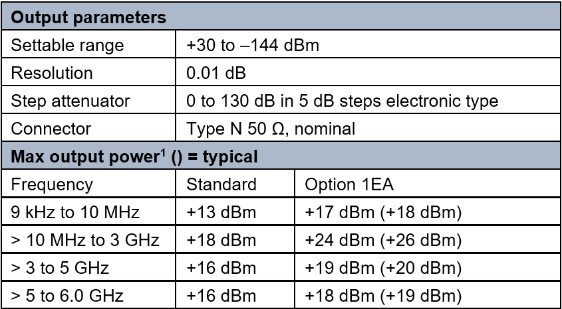Table 1. Amplitude specifications of output parameters and maximum output power

There are several points you need to be aware of when reading this table.

1. Output amplitude is affected by frequency ranges and operating temperatures.
2. An option is available for the needs of higher output power, option 1EA in this example.
3. The step attenuator (in 5-dB step) provides coarse power attenuation to achieve low power levels. Fine power level adjustment is provided by the ALC (automatic leveling control) within the attenuator hold range.
4. The “Maximum output power” is for CW. Some datasheets list maximum output power for I/Q modulation which is referring to PEP.

## Measurement Applications

If you need to go beyond the specified output power range, you can use an amplifier to increase the output power or an attenuator to decrease it. However, you will need to take the amplifier’s gain uncertainty, and the attenuator’s flatness and accuracy into consideration. Here are some test applications for high and low output power.

### High-output power test applications:

1. Overcome switching losses within automated test equipment (ATE) systems
2. Address the attenuation of signals within long cable runs
3. Over-the-air (OTA) tests
4. High-power amplifiers